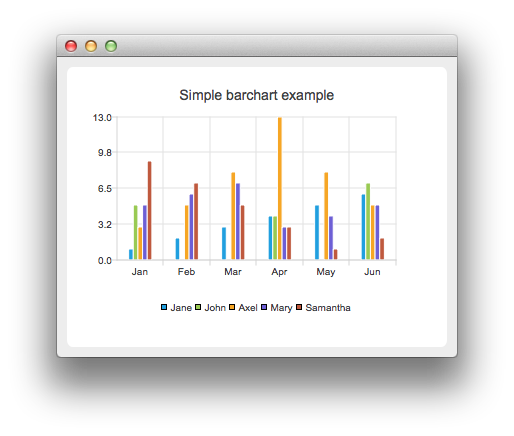# Creating Bar Charts

Note: This is part of the Charts with Widgets Gallery example.The `barsets` are used in the same way in all barcharts. To illustrate the difference between various barcharts, we use the same data in the examples. Data that the barchart visualizes, is defined by the QBarSet instances. Here we create the sets and append data to them. The data is appended here with the << operator. Alternatively, the append method could be used.

```auto set0 = new QBarSet("Jane");
auto set1 = new QBarSet("John");
auto set2 = new QBarSet("Axel");
auto set3 = new QBarSet("Mary");
auto set4 = new QBarSet("Samantha");

*set0 << 1 << 2 << 3 << 4 << 5 << 6;
*set1 << 5 << 0 << 0 << 4 << 0 << 7;
*set2 << 3 << 5 << 8 << 13 << 8 << 5;
*set3 << 5 << 6 << 7 << 3 << 4 << 5;
*set4 << 9 << 7 << 5 << 3 << 1 << 2;```

We create the series and append the barsets to it. The series takes ownership of the barsets. The series groups the data from sets to categories. First values of each set are grouped together at first category second value to second category and so on.

```QBarSeries *series = new QBarSeries;
series->append(set0);
series->append(set1);
series->append(set2);
series->append(set3);
series->append(set4);```

Here we create the chart object and add the series to it. We set the title for chart with setTitle and then turn on animations of the series by calling setAnimationOptions(QChart::SeriesAnimations)

```auto chart = new QChart;
chart->setTitle("Simple Bar Chart");
chart->setAnimationOptions(QChart::SeriesAnimations);```

To have categories displayed on axis, we need to create a QBarCategoryAxis for that. Here we create a category axis with a list of categories and set it to be aligned to the bottom, acting as the x-axis, and attach it to the series. The chart takes ownership of the axis. For y-axis we use a value axis, aligned to the left-hand side.

```QStringList categories {"Jan", "Feb", "Mar", "Apr", "May", "Jun"};
auto axisX = new QBarCategoryAxis;
axisX->append(categories);
series->attachAxis(axisX);

auto axisY = new QValueAxis;
axisY->setRange(0,15);
```chart->legend()->setVisible(true);
`createDefaultChartView(chart);`